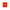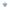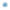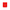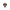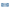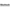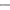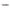# Seventh Russian-Finnish Symposium on Discrete Mathematics (RuFiDiM-2022)

## June 12 — 15, Petrozavodsk, Karelia (Russia)

The Symposium is devoted to an important and dynamically developing mathematical field — discrete mathematics. The topic of the symposium includes theoretical questions of discrete mathematics related to the problem of decidability, complexity evaluation of algorithms, automata theory, graph theory, and applications in cryptography, information networks, and other fields of computer science. This scientific event began as part of an international collaboration of Russian and Finnish scientists in discrete mathematics and related fields, but has long been an international event in terms of participants. The first Russian-Finnish Symposium on discrete mathematics was held in Russia in Saint Petersburg in 2011, and the second one in Finland in Turku in 2012. In 2014, the third Symposium was held in Petrozavodsk, the fourth Symposium was held in 2017 in Turku, the fifth Symposium was held in Veliky Novgorod in 2019, and finally the sixth Symposium will be held in Finland in 2021.

### Objectives

The seventh Symposium will discuss new results in various fields of discrete mathematics: combinatorial analysis, graph theory, automata theory, probability problems in discrete mathematics, and game-theoretic models on graphs. The Symposium will contribute to solving problems of combinatorics of words, calculating the complexity of algorithms for solving tropical linear systems, finding optimal flows in networks, job shop scheduling, and finding limit distributions of the main characteristics of random graphs. A wide range of applications in various fields will also be discussed. The best papers will be published in Lecture Notes in Computer Science (Springer) and the leading journal in this field, Fundamenta Informaticae.